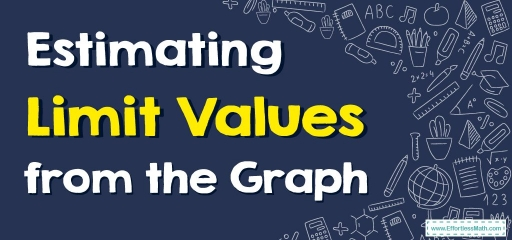# How to Estimate Limit Values from the Graph?

The best way to start reasoning about limits is by using graphs. The following step-by-step guide helps you learn how to analyze a limit graphically.Finding limits means we have to consider approximately some values of $$x$$ which are closer to the given number from the left and right sides. And check for which value of $$x$$ we are getting the function undefined. We can also check this with a graph.

## A step-by-step guide to Estimate limit values from the graph

When your pre-calculus teacher gives you the graph of a function and asks you to calculate the limits, you read values from the graph, something you’ve been doing since you first discovered what a graph was!

You follow that function from the left-hand side toward the $$x$$ value in question if you’re seeking a limit from the left.

To find the correct limit, repeat the method from the right. If the $$y$$ value approached from the left and the $$y$$ value approached from the right are the same (did the pencils meet? ), then that $$y$$ value is the limit.

Because graphing a function is a time-consuming and involved operation, you should only utilize it if you’ve been provided the graph.

### Estimating Limit Values from the Graph – Example 1:

Find the limit of $$lim_{x\to -1}f(x)$$ graphically.

You can see that as the $$x$$-value gets closer and closer to $$–1$$, the value of the function $$f(x)$$ gets $$6$$. And when $$x$$ reaches $$–1$$, the function’s value is $$6$$! However, technically, having $$f(–1) = 6$$ isn’t required to say that the limit is $$6$$; the function could have a hole in it at that point, and the limit would still be $$6$$!

### Estimating Limit Values from the Graph – Example 2:

In the above graph, find the limit of $$lim_{x\to 3}f(x)$$ graphically.

You can see a hole in the function at $$x = 3$$, which means that the function is not defined – but that does not mean you can not state a limit. If you look at the values of the function from the left, $$lim_{x\to 3^-}f(x)$$, and from the right, $$lim_{x\to 3^+}f(x)$$, you see that the $$y$$ value approaches $$3$$. So you say that the limit of the function as $$x$$ approaches $$3$$ is $$3$$.

## Exercises for Estimate Limit Values from the Graph

• Find the limit of $$lim_{x\to -2}f(x)$$ graphically.

• Find the limit of $$lim_{x\to 1}f(x)$$ graphically.
• $$\color{blue}{lim_{x\to -2}f(x)=1}$$
• $$\color{blue}{lim_{x\to 1}f(x)=5}$$

### What people say about "How to Estimate Limit Values from the Graph? - Effortless Math: We Help Students Learn to LOVE Mathematics"?

No one replied yet.

X
30% OFF

Limited time only!

Save Over 30%

SAVE $5 It was$16.99 now it is \$11.99